• 2019-07-30 17:13:53

在系统开发中，有时会用到一些常用的空间算法，引用一些类库是可以解决问题，但是有时类库的运行效率比较慢，引用的东西比较多，如果需要的方法不多，可以写一些简单的计算方法。

下边分享几个常用的gis计算方法：

//判断点是否在面里

public bool IsPointInPolygon(List<CVector> poly, CVector point)

{

int i, j;

bool c = false;

for (i = 0, j = poly.Count - 1; i < poly.Count; j = i++)

{

if ((((poly[i].VY <= point.VY) && (point.VY < poly[j].VY))

|| ((poly[j].VY <= point.VY) && (point.VY < poly[i].VY)))

&& (point.VX < (poly[j].VX - poly[i].VX) * (point.VY - poly[i].VY)

/ (poly[j].VY - poly[i].VY) + poly[i].VX))

{

c = !c;

}

}

return c;

}


//计算弧度

 public double Rad(double d)
{
return d * Math.PI / 180.0;
}


//计算角度

public static double RAngle(double d)
{
return d * 180.0 / Math.PI;
}


//计算两个坐标的中心点

 public double[] ComputeMidPoint(double lat1, double long1, double lat2, double long2)

{

var Bx = Math.Cos(lat2) * Math.Cos(long2 - long1);

var By = Math.Cos(lat2) * Math.Sin(long2 - long1);

var _rlat = Math.Atan2(Math.Sin(lat1) + Math.Sin(lat2), Math.Sqrt((Math.Cos(lat1) + Bx) * (Math.Cos(lat1) + Bx) + By * By));

var _rlong = long1 + Math.Atan2(By, Math.Cos(lat1) + Bx);

return new double[] { _rlat, _rlong };

}


//计算一批点的四至坐标

 public OCExtent GetPointsExtent(List<CVector> PList)
{

OCExtent cET = new OCExtent();

for (int i = 0; i < PList.Count; i++)

{

CVector aP = PList[i];

if (i == 0)

{

cET.minX = aP.VX;

cET.maxX = aP.VX;

cET.minY = aP.VY;

cET.maxY = aP.VY;

}

else

{

if (cET.minX > aP.VX)

{

cET.minX = aP.VX;

}

else if (cET.maxX < aP.VX)

{

cET.maxX = aP.VX;

}

if (cET.minY > aP.VY)

{

cET.minY = aP.VY;

}

else if (cET.maxY < aP.VY)

{

cET.maxY = aP.VY;

}

}

}

return cET;

}


关注公众号，多多支持！更多相关内容
• 总结常考GIS算法的步骤思路并有详细的代码实现过程，适合研究生入学数据结构或GIS原理考试
• GIS算法作业 HelloWorld.html： HelloCanvas.html： JumpHorse.html： BestWorkList.html： DrawMyName.html： DrawMyName2.html： MapProjection.html： CalculateArea.html： ...JavaScript
• GIS算法基础 》Delaunay三角网与Vrni图算法.ppt
• c# GIS算法实验系统源码实例，包括图形绘制，点选多边形，点选多段线，求多边形面积，矢量线的栅格化，矢量多边形的区域填充，点的绘制，框选点要素，通过点集构建Delaunay三角网，道格拉斯压缩， Z填充曲线的生成，...
• 第二章 GIS算法的几何基础;第二章 GIS算法的几何基础;2.1 维数扩展的9交集模型(10-1;2.1 维数扩展的9交集模型(10-2;2.1 维数扩展的9交集模型(10-3;2.1 维数扩展的9交集模型(10-4;2.1 维数扩展的9交集模型(10-5;2.1 ...
• https://github.com/XiaoZhong233/GIS_ALG/blob/master/src/scau/gz/zhw/CalculateBasic.java 目录 一、线段的拐向的判断 二、判断点是否在线段上 三、判断两线段是否相交 ①快速排斥试验 ②跨立试验 快速...

代码已经放在github上，需要的同学自取：

https://github.com/XiaoZhong233/GIS_ALG/blob/master/src/scau/gz/zhw/CalculateBasic.java

目录

一、线段的拐向的判断

二、判断点是否在线段上

三、判断两线段是否相交

①快速排斥试验

②跨立试验

快速排斥试验：

跨立试验

一、射线法的实现

转角法

二、转角法的实现

地理数据在计算机中表示大致分为两种，矢量数据栅格数据

要计算地理数据的空间关系，一般是矢量数据之间的比较。例如：点，线，面之间的比较。

如何判断线段之间是否相交，线段与面的包含关系。点与面的包含关系等等这些空间关系，都用到计算几何的算法。

空间关系的判定算法的内容有：

1、线段的拐向判断

2、判断两线段是否相交

3、判断矩形是否包含点

4、判断线段，折线，多边形是否在矩形中

5、判断矩形是否在矩形中

6、判断圆是否在矩形中

7、判断点是否在多边形内

8、判断线段是否在多边形内

9、判断折线是否在多边形内

。。。。。。等等

话不多说。一个个的来看。

# 一、线段的拐向的判断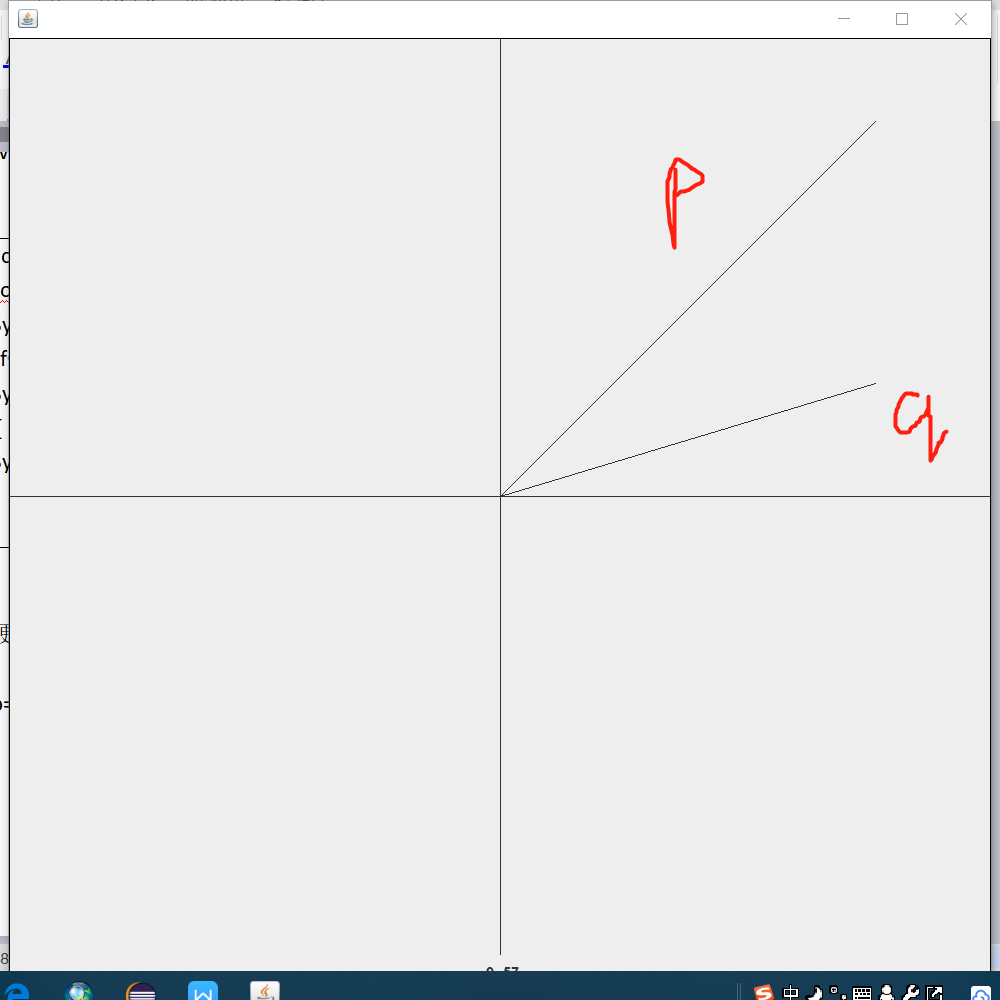现有p,q两个线段，如何判断p和q的方位问题呢？

可以利用矢量的叉积判断：

二维平面向量很的叉积很好计算 ：
例如： p=（x1,y1）,q=(x2,y2)
则 p×q=x1*y2-x2*y1;

有这么三个关系：

若PxQ>0，则说明P在Q的顺时针方向

若PxQ<0，则说明P在Q的逆时针方向

若PxQ=0，则说明PQ共线(共线有可能反向也可能正向)

	//2个向量的向量积(叉积)
public double crossProduct(Vector2D v)
{
return x * v.y - y * v.x;
}

Vector2D是我构造的工具类，用于矢量的表示

package math;

import scau.gz.zhw.Line;
import scau.gz.zhw.Point;

//平面向量(x,y)的基本运算规则,角度弧度的转换等实现
public class Vector2D {
private double x;
private double y;

public Vector2D()
{
x = 0;
y = 0;
}

public Vector2D(double _x, double _y)
{
x = _x;
y = _y;
}

/**
*
* @param a 起点
* @param b 终点
*/
public Vector2D(Point a,Point b) {
this.x=b.getX()-a.getX();
this.y=b.getY()-a.getY();
}

public Vector2D(Line line) {
this(line.getStart(), line.getEnd());
}

//获取弧度
{
return Math.atan2(y, x);
}

//获取角度
public double getAngle()
{
return getRadian() / Math.PI * 180;
}

public Vector2D clone()
{
return new Vector2D(x,y);
}

public double getLength()
{
return Math.sqrt(getLengthSQ());
}

public double getLengthSQ()
{
return x * x + y * y;
}

//向量置零
public Vector2D Zero()
{
x = 0;
y = 0;
return this;
}

public boolean isZero()
{
return x == 0 && y == 0;
}

//向量的长度设置为我们期待的value
public void setLength(double value)
{
double _angle = getAngle();
x = Math.cos(_angle) * value;
y = Math.sin(_angle) * value;
}

//向量的标准化（方向不变，长度为1）
public Vector2D normalize()
{
double length = getLength();
x = x / length;
y = y / length;
return this;
}
//是否已经标准化
public boolean isNormalized()
{
return getLength() == 1.0;
}

//向量的方向翻转
public Vector2D reverse()
{
x = -x;
y = -y;
return this;
}

//2个向量的数量积(点积)
public double dotProduct(Vector2D v)
{
return x * v.x + y * v.y;
}

//2个向量的向量积(叉积)
public double crossProduct(Vector2D v)
{
return x * v.y - y * v.x;
}

//计算2个向量的夹角弧度
//参考点积公式:v1 * v2 = cos<v1,v2> * |v1| *|v2|
public static double radianBetween(Vector2D v1, Vector2D v2)
{
if(!v1.isNormalized()) v1 = v1.clone().normalize(); // |v1| = 1
if(!v2.isNormalized()) v2 = v2.clone().normalize(); // |v2| = 1
return Math.acos(v1.dotProduct(v2));
}

//弧度 = 角度乘以PI后再除以180、 推理可得弧度换算角度的公式
//弧度转角度
{
return radian / Math.PI * 180;
}
//向量加
{
return new Vector2D(x + v.x, y + v.y);
}
//向量减
public Vector2D subtract(Vector2D v)
{
return new Vector2D(x - v.x, y - v.y);
}
//向量乘
public Vector2D multiply(double value)
{
return new Vector2D(x * value, y * value);
}
//向量除
public Vector2D divide(double value)
{
return new Vector2D(x / value, y / value);
}

public double getX() {
return x;
}

public double getY() {
return y;
}

public Line toLine() {
return new Line(new Point(0, 0),new Point(x, y));
}

public void showGUI() {
toLine().showGUI();
}

@Override
public String toString() {
// TODO Auto-generated method stub
return String.format("(%.2f,%.2f)", x,y);
}
}

判断叉积函数：

	public static void getDirection(Vector2D p,Vector2D q) {
if(p.crossProduct(q)>0) {
System.out.println("顺时针");
}else if (p.crossProduct(q)<0) {
System.out.println("逆时针");
}else {
System.out.println("共线");
}
}

测试结果：

说明：为了方便测试，我用java Swing简单写了一个GUI界面 可以绘制矢量的点，线，面，用来验证拐向函数的结果是否正确

①测试数据：p=(100,100)  q=(100,30)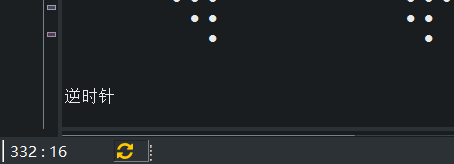②测试数据二：p=(100,100)  q=(-100,-100)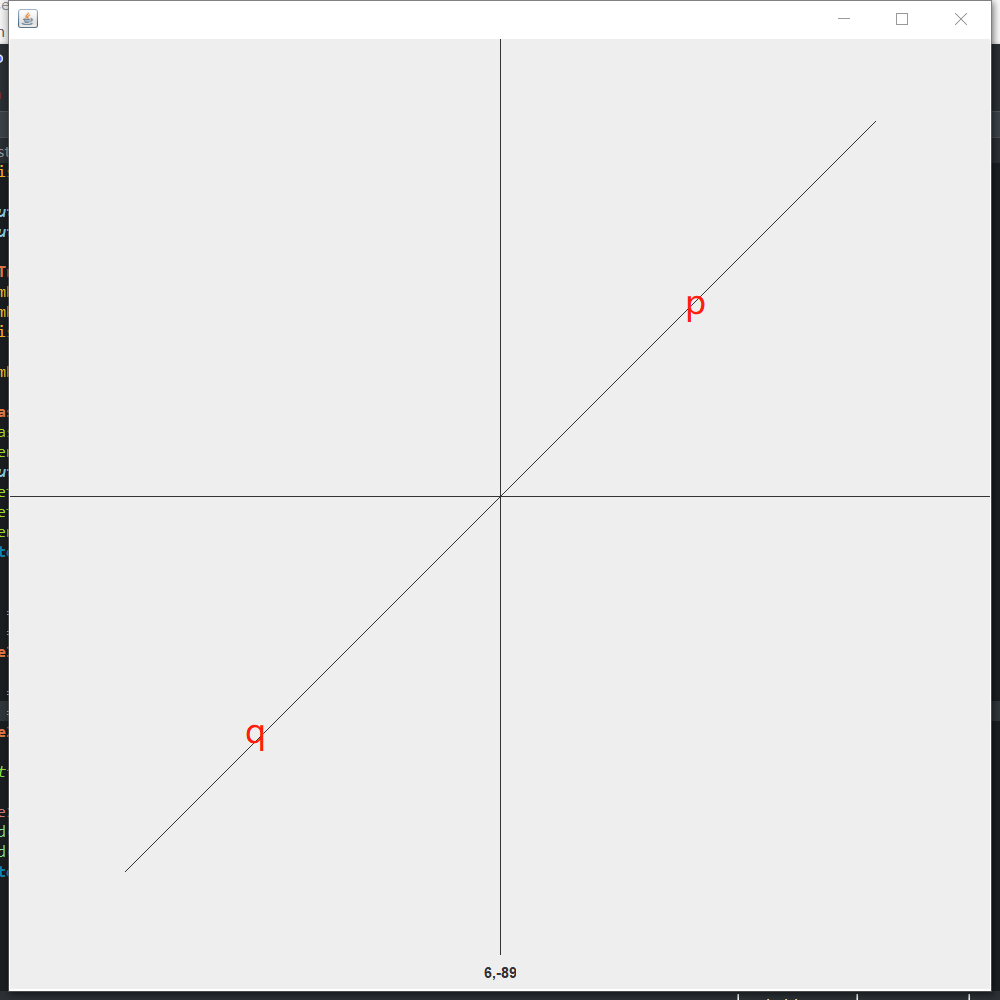控制台结果：# 二、判断点是否在线段上

设点为Q，线段为P1P2，判断点Q在该线段上的依据是（Q-P1）X(P2-1) = 0，这样就保证Q在P1P2这条直线上，但是还是不能保证在P1P2的线段上，所以我们得多加个条件：且Q在P1,P2为对角顶点的矩形内

算法：

	/**
* 判断点是否在线段上
* @param p1 线段端点
* @param p2 线段端点
* @param q	需要判断的点
* @return
*/
public static boolean isPointAtSegment(Point p1,Point p2,Point q) {

//判断点是否在线段围成的矩形区域内
if(q.getX()<=Math.max(p1.getX(), p2.getX()) && q.getX()>=Math.min(p1.getX(), p2.getX())
&& q.getY()<= Math.max(p1.getY(), p2.getY()) && q.getY()>=Math.min(p1.getY(), p2.getY()))
{
Vector2D qp1 = getVector(q, p1);
Vector2D p2p1 = getVector(p2, p1);
return qp1.crossProduct(p2p1)==0?true:false;
}else {
return false;
}

}

# 三、判断两线段是否相交

判断两线段是否相交，我的第一反应就是解方程，看两线段是否有交点，但是在GIS算法中，我们面对的是海量的数据，这样的算法因为计算量大并不高效。

因此，我们最好得有个筛选的过程，把那些明显不会相交的线段剔除掉，这样就减小了一部分的计算量。

其次，我们判断线段相交最好不要解方程，这样涉及的计算量大，可以用矢量的方法

所以，分为两步确定两天线段是否相交：

### ①快速排斥试验

设以线段a,b为对角线的矩形为R，设以线段c,d为对角线的矩形为T，如果R和T不相交，显然两线段不会相交

### ②跨立试验

如果ab,cd相交，那么ab必跨过cd，那么（ac x ab ）x（bd xab）<=0

因为ab两边一定分别有个线段（在ab顺时针方向的向量与ab的叉积小于0，在ab逆时针方向的向量与ab叉积大于0），所以乘积是小于0的。至于为什么等于0，是因为ac,和bd有可能在cd上，即ac与ab共线或bd与ab共线或ab和cd共线，那么这种情况就是等于0了，也算相交的一种情况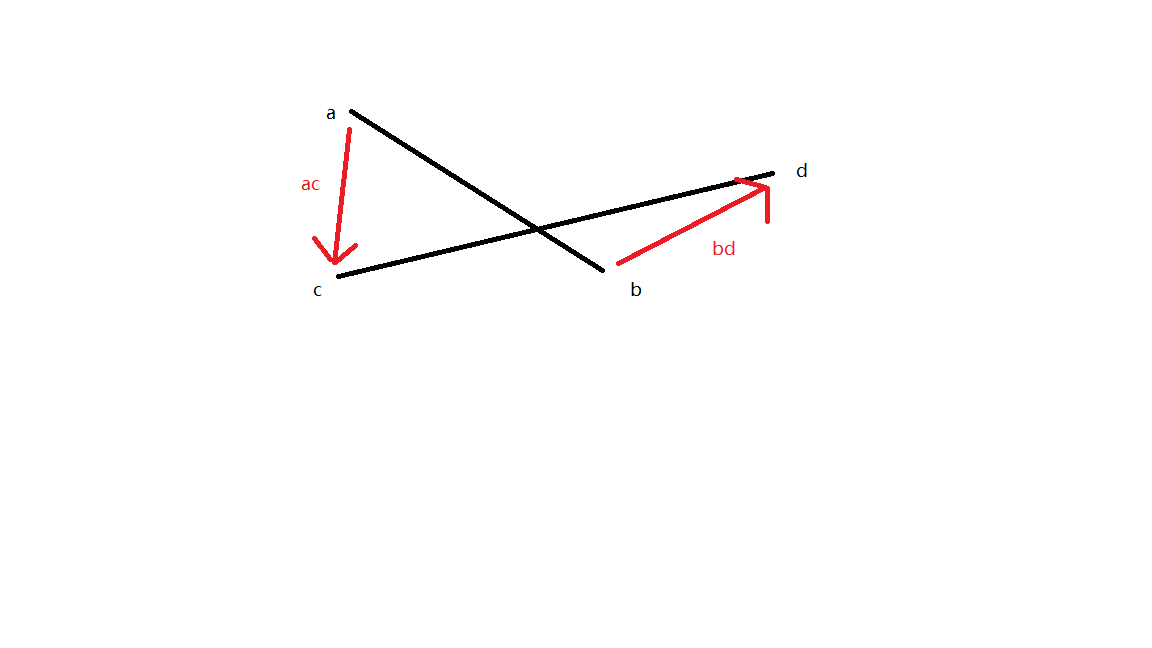## 快速排斥试验：

怎么判断两个矩形相不相交，可以这样判断：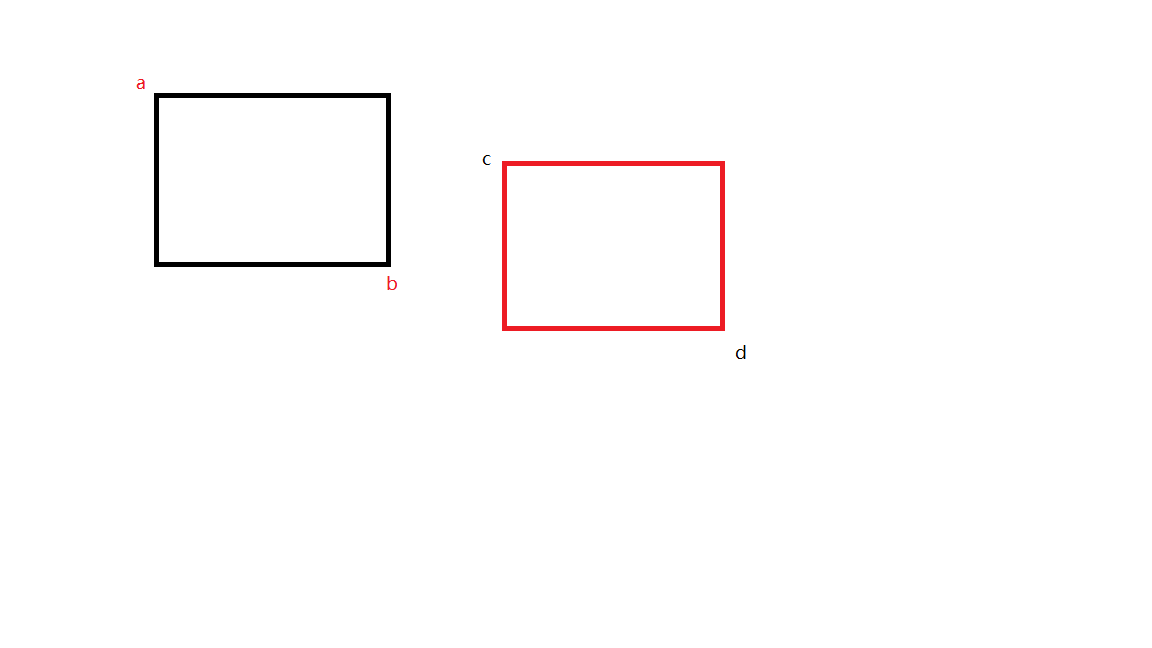①ab的较低点低于cd的较高点 （y值比较）

②cd的左端小于ab的右端(x值比较)

③cd较低点低于ab的较高点(y值比较)

④ab的左端小于cd的右端(x值比较)

算法实现：

//快速排斥试验，判断ab,cd围成的两矩形是否相交
if(Math.min(a.getY(), b.getY())<=Math.max(c.getY(), d.getY()) &&
Math.min(c.getX(), d.getX())<=Math.max(a.getX(), b.getX())&&
Math.min(c.getY(), d.getY())<= Math.max(a.getY(), b.getY()) &&
Math.min(a.getX(), b.getX())<= Math.max(c.getX(), d.getX())) {
flag1 = true;
}

## 跨立试验

//跨立试验
if(ac.crossProduct(ab) * bd.crossProduct(ab) <=0) {
flag2 = true;
}

完整算法在这：

	/**
* 判断两线段是否相交
* @param a
* @param b
* @param c
* @param d
* @return
*/
public static boolean isTwoSegmentIntersect(Point a,Point b,Point c,Point d) {
boolean flag1 = false;
boolean flag2 = false;
//快速排斥试验
if(Math.min(a.getY(), b.getY())<=Math.max(c.getY(), d.getY()) && Math.min(c.getX(), d.getX())<=Math.max(a.getX(), b.getX())
&& Math.min(c.getY(), d.getY())<= Math.max(a.getY(), b.getY()) && Math.min(a.getX(), b.getX())<= Math.max(c.getX(), d.getX())) {
flag1 = true;
}
Vector2D ab = getVector(a, b);
Vector2D ac = getVector(a, c);
Vector2D bd = getVector(b, d);
//跨立试验
if(ac.crossProduct(ab) * bd.crossProduct(ab) <=0) {
flag2 = true;
}
return flag1&&flag2;
}


三、判断点是否在多边形内

判断点是否在多边形内有两种方法，一种是射线法，另一种是转角法。

①射线法：引一条从P开始，穿过多边形边界的次数为交点数目。当交点数目为偶数时，点P在多边形外部

为方便计算选取一条水平的、从被判断点的右边延伸的，平行于x轴的射线。

为了使在某些特殊情况下判断穿越是否有效，有效穿越要符合以下几个规则：

1. 方向向上的边包括开始点，不包括终止点
2. 方向向下的边包括终止点，不包括开始点
3. 水平边不参与测试
4. 射线和多边形的边的交点必须严格在点P的右边
5. 如果点在多边形边上，则直接判断为在多边形内部（这一条可以根据不同需要设定为不同的结果）射线法算法步骤：

说明:p射线是由点p延伸出的水平x轴正方向射线

1. 开始遍历多边形的每条边
2. 判断边是否水平，如果是就跳出本次循环
3. 如果点在多边形上，直接返回true
4. 判断p射线是否在边的左边，如果不在，就直接跳过该边的计算
5. 判断p射线是否穿过边的端点，是则利用上面所述规则1,2进行穿越测试
6. 遍历结束，根据穿越数得出结果

话不多说，看算法实现吧：

## 一、射线法的实现

public static boolean isPointAtPolygon(Point[] points,Point p) {
int crossNum = 0;
boolean flag = false;
if(Double.doubleToLongBits(points[points.length-1].getX())==Double.doubleToLongBits(points.getX()) &&
Double.doubleToLongBits(points[points.length-1].getY()) == Double.doubleToLongBits((points.getY()))){
flag = true;
}

//如果最后一个点不等于第一个点
//自动闭合
ArrayList<Line> lines = new ArrayList<Line>();
if(!flag) {
for(int i=0;i<points.length;i++) {
Line line = new Line(points[i%points.length], points[(i+1)%points.length]);
}
}else {
for(int i=0;i<points.length-1;i++) {
Line line = new Line(points[i], points[i+1]);
}
}
//遍历每条边
if(type==0) {
for(Line line : lines) {
//rule#1:方向向上的边包括其开始点,不包括其终止点
//rule#2:方向向下的边包括其终止点,不包括其开始点
//rule#3:水平边不参与穿越测试
//rule#4:射线和多边形的边的交点必须严格在点p的右边

//如果点在线段上则直接判定为在多边形内部
if(isPointAtSegment(line.getStart(), line.getEnd(), p)) {
return true;
}

//rule#3
if(!line.isHorizontal()) {

//rule#4,保证p在边的右边
if(Double.doubleToLongBits(p.getX()) < Double.doubleToLongBits(line.getXByY(p.getY()))
&& Double.doubleToLongBits(line.getXByY(p.getY()))>=Double.doubleToLongBits(Math.min(line.getStart().getX(),line.getEnd().getX()))
&& Double.doubleToLongBits(line.getXByY(p.getY()))<=Double.doubleToLongBits(Math.max(line.getStart().getX(), line.getEnd().getX()))) {
//rule#1,2
//当点的射线穿过每条边的端点时，规则1,2起作用
if(Double.doubleToLongBits(p.getY()) == Double.doubleToLongBits(line.getStart().getY())
||Double.doubleToLongBits(p.getY()) == Double.doubleToLongBits(line.getEnd().getY()) ) {
if(line.isUp()) {
//判断是穿过开始点还是终止点
if(Double.doubleToLongBits(p.getY()) == Double.doubleToLongBits(line.getStart().getY())) {
//方向为上，穿过开始点，则有效穿越
crossNum++;
}else {
//方向为上，穿过终止点，则无效穿越
}
}else {
//判断是穿过开始点还是终止点
if(Double.doubleToLongBits(p.getY()) == Double.doubleToLongBits(line.getStart().getY())) {
//方向为下，穿过开始点，则无效穿越
}else {
//方向为下，穿过终止点，则有效穿越
crossNum++;
}
}
}else {
//直接计算
++crossNum;
}
}
}
}
//奇数在内，偶数在外
//System.out.println("crossNum : " +crossNum);
return crossNum%2==0?false:true;
}


注释说的很详细了，就不加赘述了。

### 转角法

转角法可以很精确地判断一个点是否在非简单多边形内部（就是多边形内部还有一些复杂的构造，后面有对比说明）。它需要计算多边形绕点有多少次。如果环绕数为零，那么点在多边形外部，非零，则点在多边形内部。

转角法也和射线法类似，遵守这么些规则：

1. 方向向上的边包括开始点，不包括终止点
2. 方向向下的边包括终止点，不包括开始点
3. 水平边不参与测试
4. 射线和多边形的边的交点必须严格在点P的右边
5. 如果点在多边形边上，则直接判断为在多边形内部（这一条可以根据不同需要设定为不同的结果）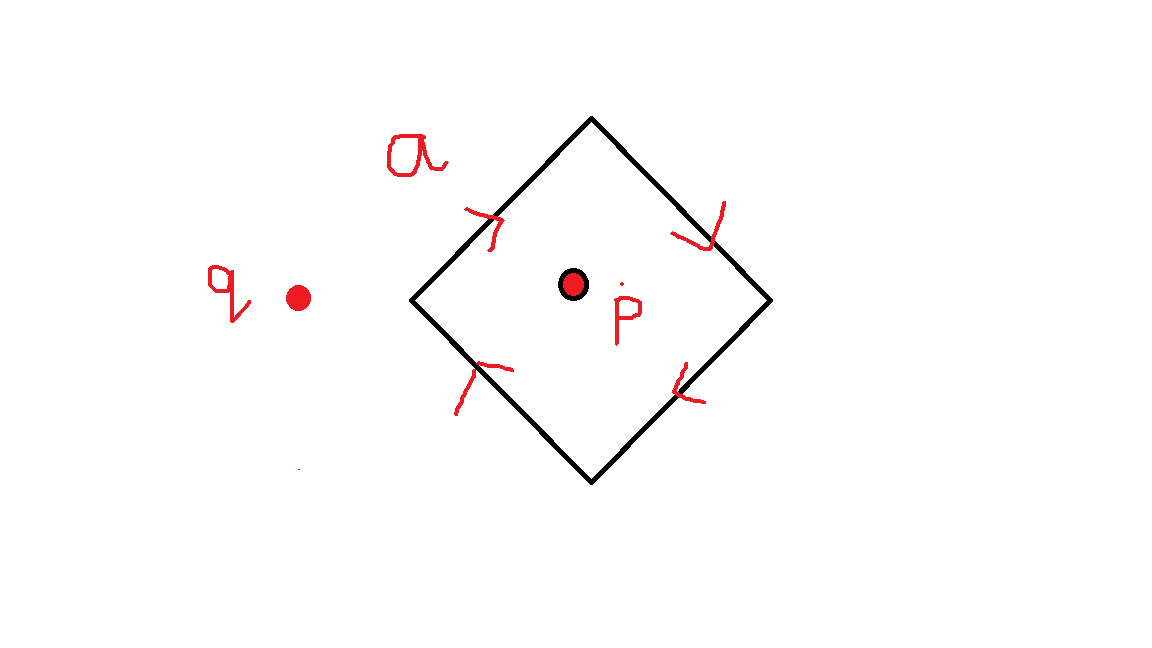看上面这个多边形，如果从多边形的a边开始遍历 （水平边不参与穿越测试），因此a跳过

规定点在边的左边（以边的前进方向为准）时环绕数+1，点在边的右边时（以边的前进方向为准）环绕数-1；

那么上边这个p点环绕数（从a边开始）的计算过程就为（-1,-1,-1,-1） 结果为-4，不等于零，说明在多边形内部

q点的环绕数计算过程（从a边开始）为（+1，-1，-1，+1），结果为0，说明在多边形外部。

关于如何判断点在边的右边还是左边，可以从点向右做一条水平射线(为了保证边与射线的交点在点的右边) 判断该射线与边的叉积即可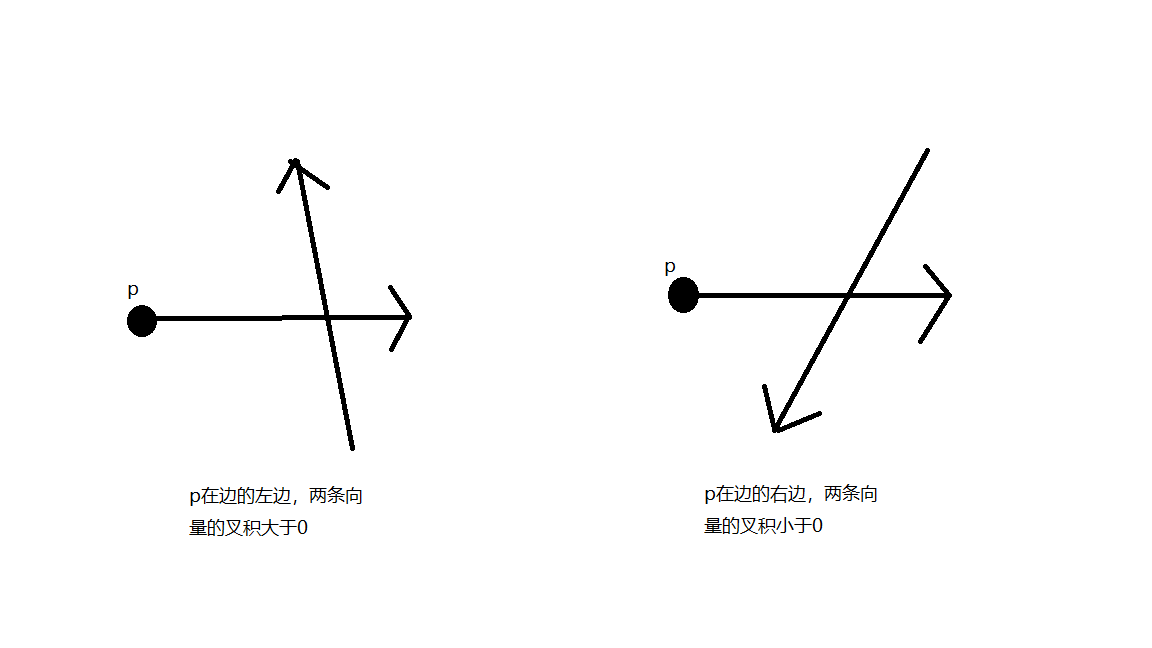## 二、转角法的实现

public static boolean isPointAtPolygon(Point[] points,Point p){
//转角法需要判断从p向右出发的水平射线与线段方向的关系，即p是否在边的左边
//int count = 0;
for(Line line:lines) {
//如果点在线段上则直接判定为在多边形内部
if(isPointAtSegment(line.getStart(), line.getEnd(), p)) {
return true;
}
if(!line.isHorizontal()) {
//构造从p出发的水平向右射线，rule#3
Vector2D pVector2d = new Vector2D(p.getX(),0);
//保证p在边的左边，rule#4
if(Double.doubleToLongBits(p.getX()) < Double.doubleToLongBits(line.getXByY(p.getY()))
&& Double.doubleToLongBits(line.getXByY(p.getY()))>=Double.doubleToLongBits(Math.min(line.getStart().getX(),line.getEnd().getX()))
&& Double.doubleToLongBits(line.getXByY(p.getY()))<=Double.doubleToLongBits(Math.max(line.getStart().getX(), line.getEnd().getX()))) {
//System.out.println("id : "+count++ +" direction: "+line.getDirection());

//边向上,p在边左边->p的向右出发的水平射线在边的顺时针方向
if(line.isUp()) {
//rule#1,2
//这种情况只在点的射线穿过每条边的端点才有用
if(Double.doubleToLongBits(p.getY()) == Double.doubleToLongBits(line.getStart().getY())
||Double.doubleToLongBits(p.getY()) == Double.doubleToLongBits(line.getEnd().getY()) ) {
//判断是穿过开始点还是终止点
if(Double.doubleToLongBits(p.getY()) == Double.doubleToLongBits(line.getStart().getY())) {
//方向为上，穿过开始点，则有效穿越
if(pVector2d.crossProduct(line.getVector2D())>0) {
crossNum++;
}else {
crossNum--;
}
}else {
//方向为上，穿过终止点，则无效穿越
}
}else {
//不穿过端点的情况
if(pVector2d.crossProduct(line.getVector2D())>0) {
crossNum++;
}else {
crossNum--;
}
}

}
//边向下,p在边左边->p的向右出发的水平射线在边的顺时针方向
if (line.isDown()) {
//rule#1,2
//这种情况只在点的射线穿过每条边的端点才有用
if(Double.doubleToLongBits(p.getY()) == Double.doubleToLongBits(line.getStart().getY())
||Double.doubleToLongBits(p.getY()) == Double.doubleToLongBits(line.getEnd().getY()) ) {
//判断是穿过开始点还是终止点
if(Double.doubleToLongBits(p.getY()) == Double.doubleToLongBits(line.getStart().getY())) {
//方向为下，穿过开始点，则无效穿越
}else {
//方向为下，穿过终止点，则有效穿越
if(pVector2d.crossProduct(line.getVector2D())>0) {
crossNum++;
}else {
crossNum--;
}
}
}else {
//不穿过端点的情况
if(pVector2d.crossProduct(line.getVector2D())>0) {
crossNum++;
}else {
crossNum--;
}
}
}
}
}
}

上面也说到，转角法更为精确一些，为什么呢？

因为在一些多边形中可能会有复杂的构造，这种情况下，射线法的计算结果会不准确

例如：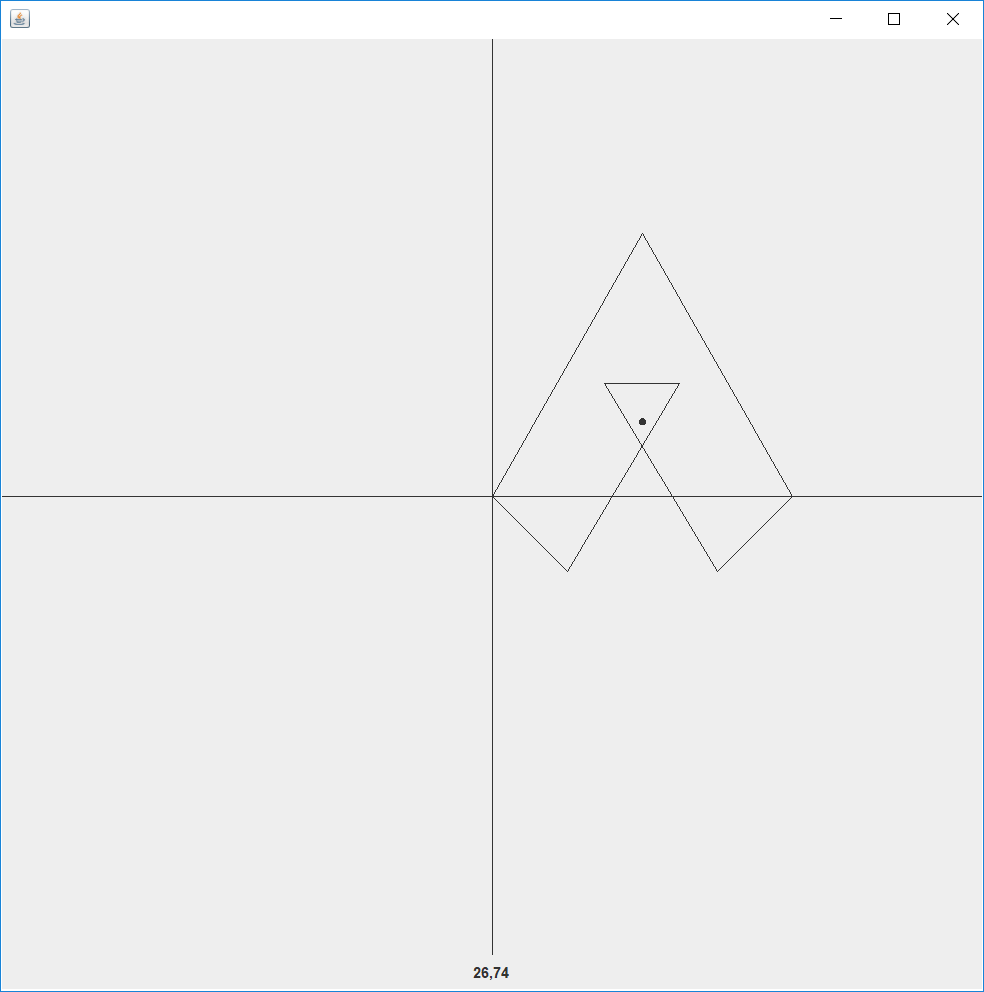这种情况下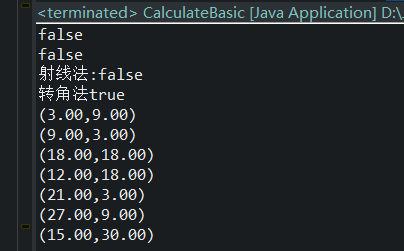可以看到同样的多边形，同样的点，用不同的方法会得到不同的结果。这是因为转角法更精确，而射线法的缺点在于没有考虑到多边形内部的复杂构造可能使结果出现偏差。

转角法是在射线法的基础上进行的优化，他具有和射线法相同的效率，并且，它更为精确，因此，判断点是否在任意多边形时，转角法是首选

射线法的思路比较简单，只需要判断穿越次数的奇偶性即可。但需要注意的是，判断穿越为有效穿越需要严格遵守4个规则。但是射线法的缺陷也是很明显的，它的判断不够精确。在左图的情况下，射线法的结果是false，而转角法的结果是true。

射线法是没有考虑到多边形内部的构造的。而转角法是射线法的优化（因为他们使用了相同的穿越规则），它通过计算环绕次数来得出结果，会考虑多边形内部的构造问题。

在同样的效率下，转角算法比射线算法准确度更高。

同时，我认为这也是个仁者见仁智者见智的过程，因为不同的场景下，可能把多边形形成的内部构造的认为是属于该多边形的，也可能认为是不属于的。因此具体的使用要根据具体场景而选择不同的方法。

展开全文• C#编写的投影变换，包括墨卡托、兰伯特投影，以及道格拉斯压缩，左转算法，线性四叉树等综合在一起的GIS算法基础演示程序，点击主窗口上的对应功能按钮，会打开相应的子程序演示窗口，在窗口内按要求操作，演示相应...
• 上一节中我们详细的介绍了什么是最大熵模型，也推导出了最大熵模型的目标公式，但是没给出如何求解的问题，本节将详细讲解GIS算法求解最大熵模型的过程，这里先把上一节的推导出的公式拿过来： 上面第一个式子是...

上一节中我们详细的介绍了什么是最大熵模型，也推导出了最大熵模型的目标公式，但是没给出如何求解的问题，本节将详细讲解GIS算法求解最大熵模型的过程，这里先把上一节的推导出的公式拿过来：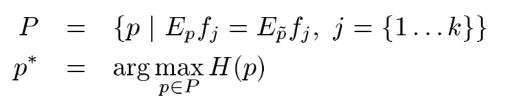上面第一个式子是说我们要寻找的P要满足k个约束条件，下式说是在满足的约束的情况下，找到是熵值最大的那个P。下面给出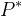的形式：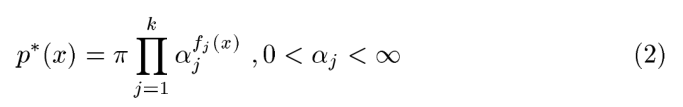上式是指数族表达式，只有一个未知数即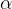，其中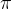是归一化因子，一旦约束条件确定，那么归一化因子就确定了，为什么要有归一化因子呢？因为总概率和为1这个条件必须得满足的。所以现在我们需要求出（2）的未知数即，怎么求呢？需要通过GIS算法，下面就详细的介绍GIS算法。

# GIS算法（Generalized Iterative Scaling）

本节还是参考那篇文章即《A Simple Introduction to Maximum Entropy Models for Natural Language Processing》，大家可以对照看一下，下面正式开始本节的内容：

首先我们先使所有特征函数的和等于C，如下：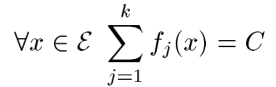那可能就有人怀疑了，这个C怎么确定才能满足呢？这里给出一种可能：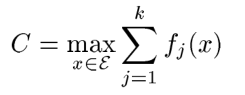既然取得是最大的，那么就有可能不满足上式，怎么办呢？这里引入一个矫正函数，和上面的形式一样，怎么确定这个矫正函数呢？如下所示：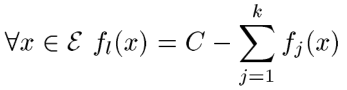上式挺暴力的，如果不满足，我把剩下的直接付给一个函数，然后在加上去就满足了，确实满足了。其实这样做对后面的计算没什么影响，我们继续往下看;

这里我们需要先假设至少存在一个特征函数为1，目的是总概率必须为1，如下：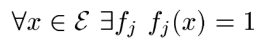下面我们就看看如何求解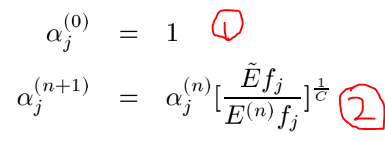其中：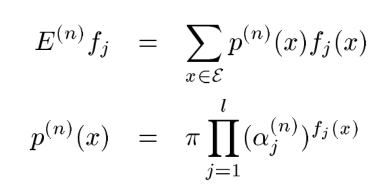如①式第一次迭代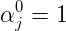说明刚开所有的特征的概率相等，此时的熵是最大的，这个可以为1 ，那么上面的第②式子就是迭代了，其实第二个式子很简单，大家不要把他们理解的太难了，我们一起来看看他是如何迭代的， 其中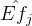是原始的特征期望或者说是约束条件的和，而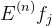是第n次迭代得到的期望，那么他们的比值说明了什么呢，如果第n次迭代的结果偏大即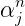偏大，则比值会小于1，那么乘上小于1的数就会使得第n+1的值减小即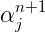小，这样就会不断的向着最优解靠近了，和EM的思想差不多，这里大家需要好好理解。

GIS的大概思路就是这样的，但是这个算法虽然可以求解我们的最大熵模型，但是计算量太大了，以至于使用受限，计算量主要体现在哪里呢？计算量主要在自然语言处理的特征很多的，有时一个文本中的特征有上百万个，而每个特征都要参与上面的计算，而且还要不停的多次迭代，这就导致了计算量很大的原因，因此这个算法的使用受到了限制，那如何改进呢？后来
Vincent J. Della Pietra  提出了改进型的迭代算法即IIS算法（Improved Iterative Scaling），他是如何改进计算量的呢？我们下面看看：

# IIS算法（Improved Iterative Scaling）

其实IIS和GIS很类似，不同之处在于GIS算法有一个矫正项，目的是使的所有特征特征等于一个常数C，但是并没有那么容易满足，因此IIS的和他不同之处就是我可以不用非要满足这个所有特征等于一个常数，如果等于C就按照GIS进行求解，如果不满足就按照牛顿法进行求解即可。下面直接给出原始论文的IIS算法过程，当然看李航的书也是一样的，只是写的方式有点不一样，论文里是使用表示指数族，而李航的未知数直接放在以e为底的指数上了，大家需要注意区别，同时IIS算法出自这篇论文

《A Maximum Entropy Approach to Natural Language Processing》Vincent J. Della Pietra ，下面给出这篇论文的算法过程：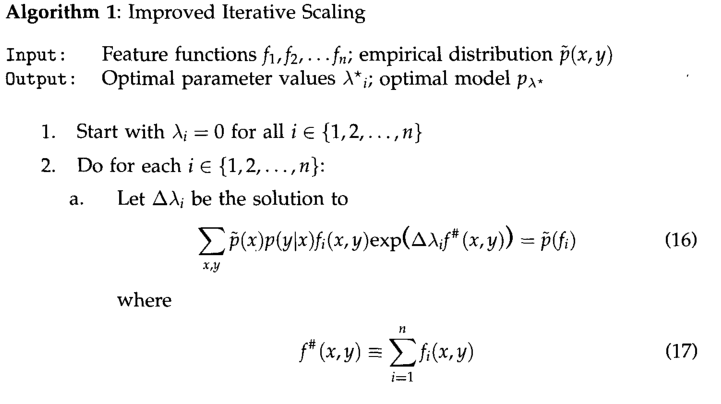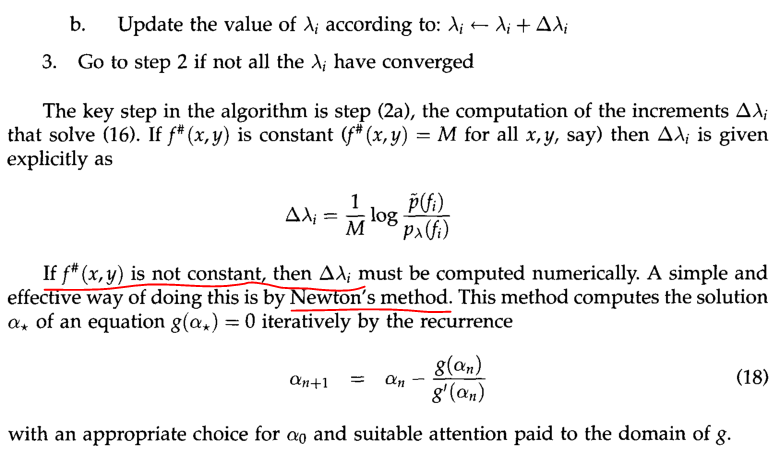这里建议先看看李航先生的书即《统计学习方法》的第六章的最大熵模型，如果大家对上一节的原理搞明白了，那么看他的书还是很容易理解的，只是使用的表达式不一样吧了，虽然都是指数族函数，但是他那本书使用的是以自然对数的指数族函数，原理和我们上一节是一样的，只是求解最大熵模型的思路不一样，我们上面使用的是GIS算法进行求解，李航先生的书是根据拉格朗日乘子进行求解的，其实他的主要思路是我们上一节不是有两个约束条件吗，如果大家理解支持向量机的话，理解他的解决思路就很简单了，这里不懂的建议还是系统的把支持向量机学习一下吧，我在机器学习模块中对此进行了详细的讲解，这里大家可以去看看。他把含有约束条件的求解最大熵模型通过拉格朗日乘子法，把约束条件和目标式子合二为一，通过对偶问题进行求解，最后就得到一个最大的概率含参数的模型，通过样本就可以求出参数，最后就可以确定了参数的值，这就是李航的解法。后面李航的书继续介绍了最优化算法，即IIS算法，讲解的很详细，这里就不细讲了，也不做搬运工了，大家可以找到这本书好好研究，当然需要你有很好的数学基础，如果你对SVM的理解很深入，我觉的看他的书是一种享受，他写的很简明扼要，里面会有大量的数学公式，看起来也很顺利，这里简单的把IIS算法的过程讲解一下，细节部分，建议看李航的书。

已知最大熵模型为：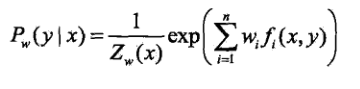其中：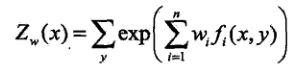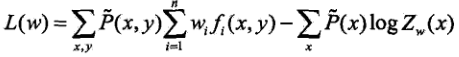上式L(w)就是通过拉格朗日乘子法最后确定的模型，通过极大释然估计求得w就求出了最大熵模型的参数了。

详细的推倒过程请查看李航的书，这里不再赘述了。请大家一定要好好看看那一章，一定要深入理解他们，这里我就偷懒了，不再叙述了。下面简单的介绍一下把最大熵模型和隐马尔可夫结合在一起的应用。

# MEMM（maximum-entropy Markov model,MEMM）

最大熵马尔可夫模型(maximum-entropy Markov model，MEMM）又称条件马尔可夫模型（conditionalMarkovmodel，CMM)，由Andrew McCallum，DayneFreitag和FernandoPereira二人于2000年提出[McCallum，2000〕。它结合了隐马尔可夫模型和最大熵模型的共同特点，被广泛应用于处理序列标注问题。文献[McCallumal.，2000]认为，在HMM模型中存在两个问题：．在很多序列标注任务中，尤其当不能枚举观察输出时，需要用大量的特征来刻画观察序列。如在文本中识别一个未见的公司名字时，除了传统的单词识别方法以外，还需要用到很多特征信息，如大写字母、结尾词、词性、格式、在文本中的位置等。也就是说，我们需要用特征对观察输出进行参数化。(2)在很多自然语言处理任务中，需要解决的问题是在已知观察序列的情况下求解状态序列，HMM采用生成式的联合概率模型（状态序列与观察序列的联合概率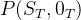来求解这种条件概率问题，这种方法不适合处理用很多特征描述观察序列的情况。为此，MEMM直接采用条件概率模型，从而使观察输出可以用特征表示，借助最大熵框架进行特征选取。

MEMM主要求解HMM的第二个问题，因此这里我们看看他们二者的区别：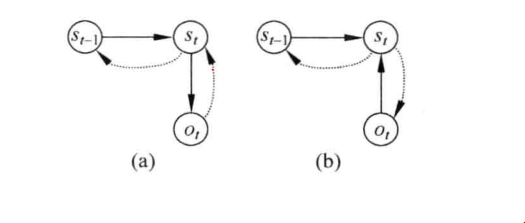（a）为传统HMM的依存关系图，实线箭头表示所指的结点依赖于箭头起始结点，虚线箭头表示箭头所指的结点是起始结点条件。图（b）为MEMM的依存关系图。在HMM中解码过程求解的是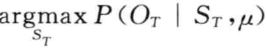，而在MEMMM中解码器

求解的是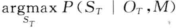。在HMM中，当前时刻的观察输出只取决于当前状态，而在MEMM中，当前时刻的观察输出还可能取决于前一时刻的状态。假设已知观察序列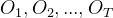研，要求解状态序列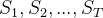，并使条件概率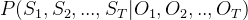最大。在MEMM中，将这一概率因子化为马尔可夫转移概率，该转移概率依赖于当前时刻的观察和前一时刻的状态：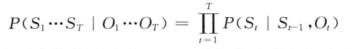对于前一时刻每个可能的状态取值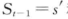的概率通过最大熵分类器建模和当前观察输出，当前状态取值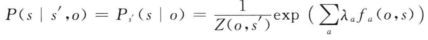其中，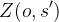为归一化因子，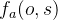为特征函数，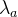为特征函数的权重，可以利用GIS算法，从训练样本中估计出来。可以通过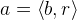定义，其中，b是当前观察的{0，1}二值特征，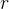是状态取值。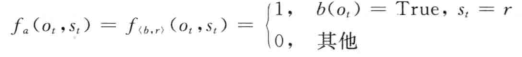HMM中用于参数估计的Baum-Welch算法修改后可用于MEMM的状态转移概率估计。在Viterbi算法中，如果t时刻到达状态5时产生观察序列的前向概率为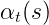,那么，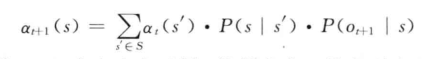在MEMM中，将定义为在时刻t的状态为s,给定到达t时刻为止的观察序列时的前向概率：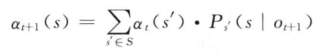相应的后向概率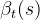为在给定t时刻之后的观察序列时，从t时刻、状态开始的概率：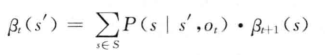MEMM是有向图和无向图的混合模型，其主体还是有向图框架。与HMM相比，MEMM的最大优点在于它允许使用任意特征刻画观察序列，这一特性有利于针对特定任务充分利用领域知识设计特征。MEMM与HMM和条件随机场(conditional random fields，CRFs)模型相比，MEMM的参数训练过程非常高效，在HMM和CRF模型的训练中，需要利用前向后向算法作为内部循环，而在MEMM中估计状态转移概率时可以逐个独立进行。MEMM的缺点在于存在标记偏置问题（labelbiasproblem)，其中一个原因是熵低的状态转移分布会忽略它们的观察输出，而另一个原因是MEMM像HMM一样，其参数训练过程是自左向右依据前面已经标注的标记进行的，一旦在实际测试时前面的标记不能确定时，MEMM往往难以处理。

下一节我们在这个基础上进行引入条件随机场。

展开全文• java、python、js都有可以引用的第三方包，实现GIS的空间算法。 java是jts，python是shapely，js是turf。 其中jts值得首先拥有，因为jts提供了一个界面工具JTS TestBuilder，可以在上面绘制图形，验证各种算法。 ...

java、python、js都有可以引用的第三方包，实现GIS的空间算法。

java是jts，python是shapely，js是turf。

其中jts值得首先拥有，因为jts提供了一个界面工具JTS TestBuilder，可以在上面绘制图形，验证各种算法。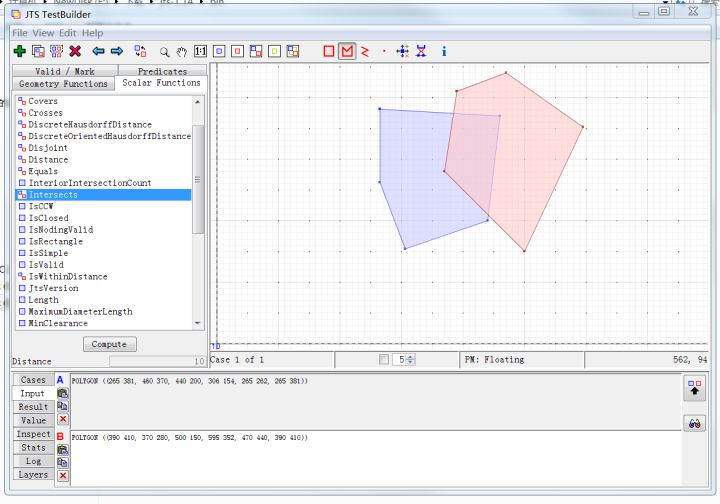下载后，将压缩包解压，在jts-1.14文件夹下的bin文件夹中，双击testbuilder.bat，既可启动JTS TestBuilder。

举一个最简单的例子，看JTS TestBuilder的使用方法。

判断两个面是否相交。

1.首先点击工具条上的Draw Polygon，然后进入侧边栏，点击input，点击A输入框，在图板上绘制图形，双击结束绘制。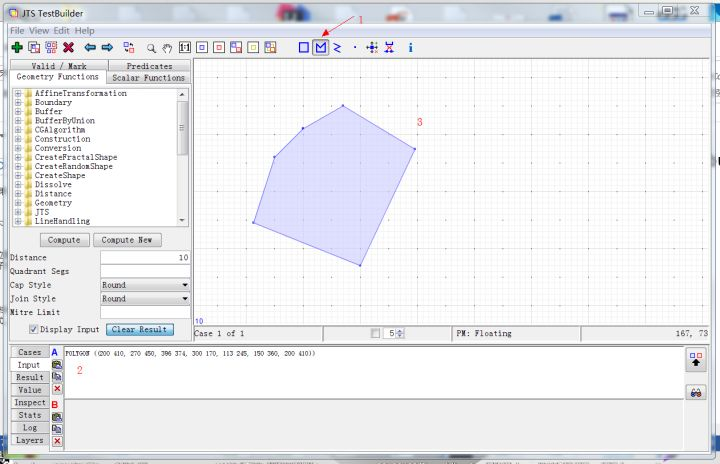2.点击input的B输入框，切换到绘制图形B，绘制完成后，双击结束。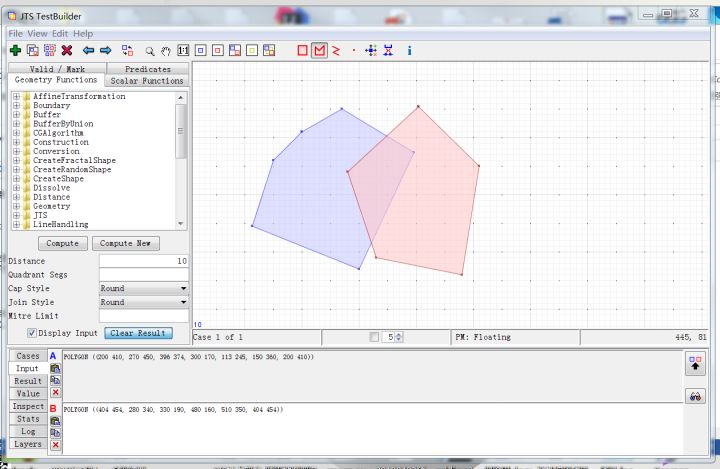3.在Scalar Functions中，选择Intersects，点击compute，下面控制台中输出结果，Value of: Intersects 耗时18ms，结果为true，两个图形相交。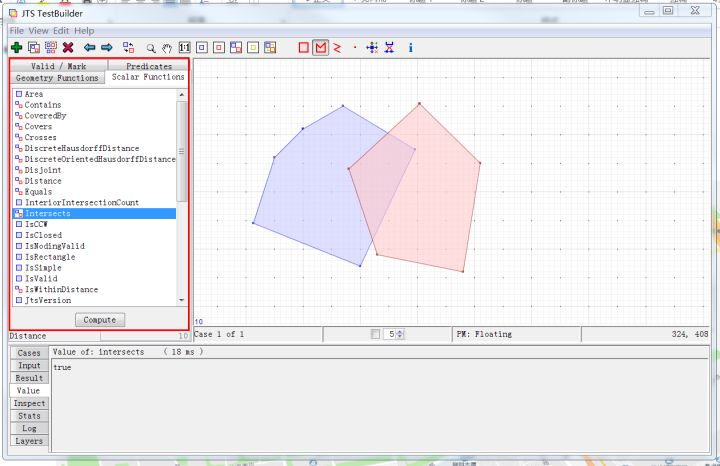工具中Valid/Mark用于判断几何图形是否合法简单；Predicates获取DE-9IM模型结果；Scalar Function查验几何图形的空间关系；Geometry Functions对几何对象进行仿射、缓冲等操作，并获取结果。

展开全文JTS 可视化工具 Java
• (但这不与学一些矢量、图像处理算法冲突，可以试着用MATLAB、OpenCV处理图像，用GDAL、shapely处理矢量，与软件处理结果进行对比分析。)做地理空间分析，要会用软件，善用软件，如ARCGIS和QGIS等，且ARCGIS和QGIS都...
• 最近在学习GIS算法，在学习过程中，想把一些经典的算法或者思想记录下来，分享给大家   计算几何基础本来是计算机图形学的内容，但是GIS在图像处理中是离不开计算机处理的，所以GIS算法基础第一个应该是计算几何...
• 公司项目中用到的一些GIS算法，记录一下。所有经纬度都是基于WGS-84标准，为Google Earth上的经纬度。 1.已知两个点的经纬度，求它们的直线距离 python代码实现 from math import * def getDistance(latA, lonA, ...
• 地形算法，描述地理全貌，山形，点、角度、方位等算法详解
• 面状换的射线算法已经放在github上：目录一、常见的面转换算法面状矢量数据是由闭合的线段组成的，在向栅格数据转换的过程中，可以先把边界做线状栅格化(线状栅格化的方法在算法基础)(五)中已经实现)，剩下的工作...
• 这是为GIS算法教学服务开发的一个小型GIS系统。
• 最大熵模型GIS算法的Python实现 最大熵模型工具包
• 矩形相关的算法 算法 方法 判断矩形是否包含点 判断该点的横坐标和纵坐标是否夹在矩形的左右边和上线边之间 判断线段、折线、多边形是否在矩形中 矩形是个凸集 =&gt; 判断所有端点是否都在矩形中 ...
• 文章目录1、常用算法1.1、计算两经纬度点之间的距离1.2、根据已知线段以及到起点距离，求目标点坐标1.3、已知点、线段，求垂足1.4、线段上距离目标点最近的点1.5、点缓冲1.6、点和面关系1.7、线段与线段的关系1.8、...javascript
• 挺不错的gis算法讲解，讲解很细，适合初学者。有想学习的朋友下载。
• GIS算法所需要一些常用工具，gdal 工具，文件解析工具，log，等等
• GIS算法基础——左转算法拓扑生成基于JavaScript的左转算法拓扑生成拓扑生成算法的技术路线弧段预处理左转算法流程构建结点、弧段、多边形类左转算法部分匹配多边形岛可视化效果总结 基于JavaScript的左转算法拓扑...javascript
• GIS算法中我们最常用的算法计算，面和面是否相交，线段之间是否相交，今天我就分享一个直线或线段与线段相交并求其交点的算法。 基本思路： 如图：（请忽略我的作图水平） 1. 则先判断L0和L1是否相交，如图...
• Kriging是地统计学的重要内容之一,是建立在变异函数理论及结构分析基础之上的 Kriging适用的条件是如果变异函数和相关分析的结果表明区域化变量存在空间相关性 三四变异函数协方差函数 半变异值的变化随着距离的加大......